# Nonconstant-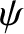Limit

Suppose that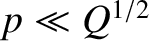. In this limit, Equation (5.78) reduces to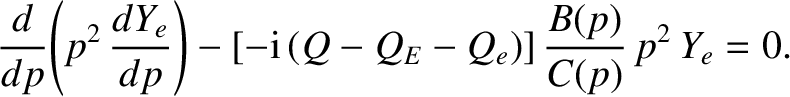(5.99)

In the various nonconstant-regimes considered in Section 5.11, the previous equation takes the form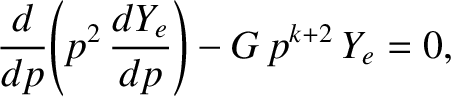(5.100)

where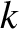is real and non-negative, and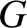is a complex constant. Let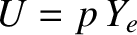. The previous equation yields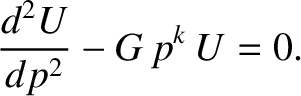(5.101)

This equation is identical in form to Equation (5.88), which we have already solved. Indeed, the solution that is bounded as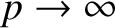has the small-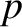expansion (5.90), where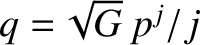,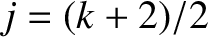, and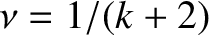. Matching to Equation (5.83) yields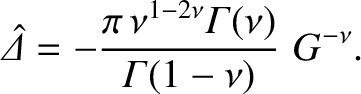(5.102)

The layer width in-space again scales as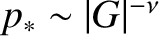. This width must be less that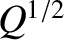. As before, the neglect of the term involving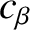in Equation (5.74) is justified provided that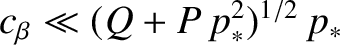.